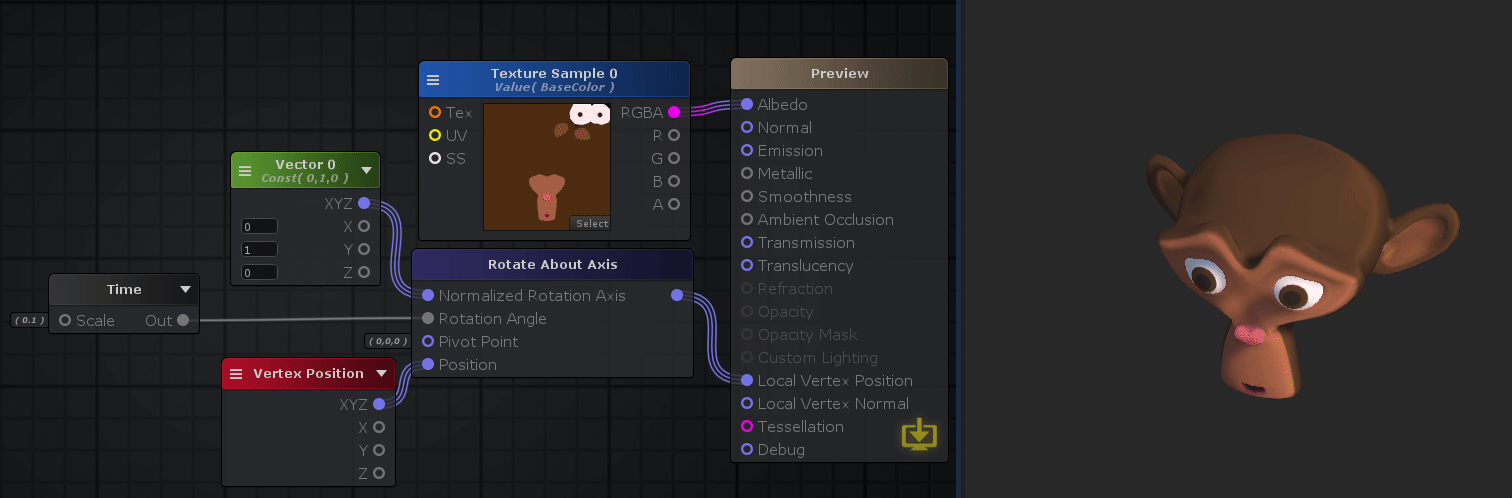The Rotate About Axis node rotates an object on a certain amount according to a specified axis and pivot point.Nodes used: Texture Sample, Vector3, Time, Vertex Position, Rotate About Axis

Node Parameter Description Default Value
Normalized Rotation Axis Axis on which the object will rotate. Only visible if the respective input port is not connected. (0,0,0)
Rotation Angle Amount of rotation to be applied. Only visible if the respective input port is not connected. 0
Pivot Point Pivot point from which the rotation will be applied and can be also seen as where is the center of the object. Only visible if the respective input port is not connected. (0,0,0)
Position Current vertex position to apply the rotation. Only visible if the respective input port is not connected. (0,0,0)
Normalize Axis Makes sure that the specified axis is a normalized vector by applying a Normalize operation on it. Off

Input Port Description Type
Normalized Rotation Axis Axis on which the object will rotate. Float3
Rotation Angle Amount of rotation to be applied. Float
Pivot Point Pivot point from which the rotation will be applied. Can be also seen as where is the center of the object. Float3
Position Current vertex position to apply the rotation. Float3